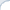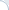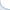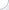This is written in response to a Quora question, which asks about improving the efficiency of machine learning models without increasing hardware capacity. Feel free to vote there for my answer on Quora!

Efficiency in machine learning in general and deep learning in particular is a huge topic. Depending on what is the goal, different tricks can be applied.

1. If the model is too large, or you have an ensemble, you can train a much smaller student model that mimics behavior of a large model. You can train to predict directly the probability distribution (for classification). The classic paper: "Distilling the Knowledge in a Neural Network" by Hinton et al., 2015.

2. Use a simpler model and/or smaller model, which parallelizes well. For example, one reason transformer neural models are effective is that they are easier/faster to train compared to LSTMs.

3. If the model does not fit into memory, you can train it using mixed precision: "Mixed precision training" by Narang et al 2018.

4. Another trick, which comes at the expense of run-time, consists in discarding some of the tensors during training and recomputing them when necessary: "Low-Memory Neural Network Training: A Technical Report" Sohoni et al, 2019. There is a Google library for this: "Introducing GPipe, an Open Source Library for Efficiently Training Large-scale Neural Network Models."

5. There is a tons of work on quantization (see, e.g., Fixed Point Quantization of Deep Convolutional Networks" by Lin et al 2016) and pruning of neural networks ("The Lottery Ticket Hypothesis: Finding Sparse, Trainable Neural Networks" by Frankle and Carbin.) I do not remember a reference, but it is possible to train quantized models directly so that they use less memory.